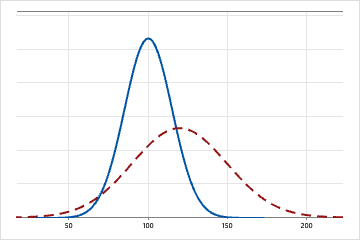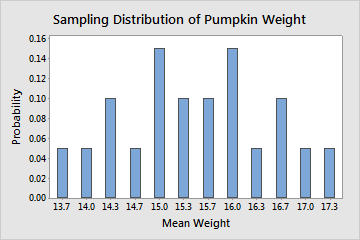# What are parameters, parameter estimates, and sampling distributions?

When you want to determine information about a particular population characteristic (for example, the mean), you usually take a random sample from that population because it is infeasible to measure the entire population. Using that sample, you calculate the corresponding sample characteristic, which is used to summarize information about the unknown population characteristic. The population characteristic of interest is called a parameter and the corresponding sample characteristic is the sample statistic or parameter estimate. Because the statistic is a summary of information about a parameter obtained from the sample, the value of a statistic depends on the particular sample that was drawn from the population. Its values change randomly from one random sample to the next one, therefore a statistic is a random quantity (variable). The probability distribution of this random variable is called sampling distribution. The sampling distribution of a (sample) statistic is important because it enables us to draw conclusions about the corresponding population parameter based on a random sample.

For example, when we draw a random sample from a normally distributed population, the sample mean is a statistic. The value of the sample mean based on the sample at hand is an estimate of the population mean. This estimated value will randomly change if a different sample is taken from that same normal population. The probability distribution that describes those changes is the sampling distribution of the sample mean. The sampling distribution of a statistic specifies all the possible values of a statistic and how often some range of values of the statistic occurs. In the case where the parent population is normal, the sampling distribution of the sample mean is also normal.

The following sections provide more information on parameters, parameter estimates, and sampling distributions.

Parameters are descriptive measures of an entire population that may be used as the inputs for a probability distribution function (PDF) to generate distribution curves. Parameters are usually signified by Greek letters to distinguish them from sample statistics. For example, the population mean is represented by the Greek letter mu (μ) and the population standard deviation by the Greek letter sigma (σ). Parameters are fixed constants, that is, they do not vary like variables. However, their values are usually unknown because it is infeasible to measure an entire population.

Each distribution is entirely defined by several specific parameters, usually between one and three. The following table provides examples of the parameters required for three distributions. The parameter values determine the location and shape of the curve on the plot of distribution, and each unique combination of parameter values produces a unique distribution curve.
Distribution Parameter 1 Parameter 2 Parameter 3
Chi-square Degrees of freedom
Normal Mean Standard deviation
3-Parameter Gamma Shape Scale Threshold
For example, a normal distribution is defined by two parameters, the mean and standard deviation. If these are specified, the entire distribution is precisely known.The solid line represents a normal distribution with a mean of 100 and a standard deviation of 15. The dashed line is also a normal distribution, but it has a mean of 120 and a standard deviation of 30.

## About parameter estimates (also called sample statistics)

Parameters are descriptive measures of an entire population. However, their values are usually unknown because it is infeasible to measure an entire population. Because of this, you can take a random sample from the population to obtain parameter estimates. One goal of statistical analyses is to obtain estimates of the population parameters along with the amount of error associated with these estimates. These estimates are also known as sample statistics.

There are several types of parameter estimates:
• Point estimates are the single, most likely value of a parameter. For example, the point estimate of population mean (the parameter) is the sample mean (the parameter estimate).
• Confidence intervals are a range of values likely to contain the population parameter.

For an example of parameter estimates, suppose you work for a spark plug manufacturer that is studying a problem in their spark plug gap. It would be too costly to measure every single spark plug that is made. Instead, you randomly sample 100 spark plugs and measure the gap in millimeters. The mean of the sample is 9.2. This is the point estimate for the population mean (μ). You also create a 95% confidence interval for μ which is (8.8, 9.6). This means that you can be 95% confident that the true value of the average gap for all the spark plugs is between 8.8 and 9.6.

A sampling distribution is the probability distribution of a given statistic, such as the mean. To illustrate a sampling distribution, let's examine a simple example where the complete population is known. For example, the following table shows the weights of the entire population of 6 pumpkins. The pumpkins can only be one of the weight values listed in the following table.
 Pumpkin 1 2 3 4 5 6 Weight 19 14 15 12 16 17

Even though the entire population is known, for illustrative purposes, we take all possible random samples of the population that contain 3 pumpkins (20 random samples). Then, we calculate the mean of each sample. The sampling distribution for the sample mean is described by all the sample means for every possible random sample of 3 pumpkins, which is shown in the following table.

Sample Weights Mean Weight Probability
2, 3, 4 14, 15, 12 13.7 1/20
2, 4, 5 14, 12, 16 14 1/20
2, 4, 6 14, 12, 17 14.3 2/20
3, 4, 5 15, 12, 16
3, 4, 6 15, 12, 17 14.7 1/20
1, 2, 4 19, 14, 12 15 3/20
2, 3, 5 14, 15, 16
4, 5, 6 12, 16, 17
2, 3, 6 14, 15, 17 15.3 2/20
1, 3, 4 19, 15, 12
1, 4, 5 19, 12, 16 15.7 2/20
2, 5, 6 14, 16, 17
1, 2, 3 19, 14, 15 16 3/20
3, 5, 6 15, 16, 17
1, 4, 6 19, 12, 17
1, 2, 5 19, 14, 16 16.3 1/20
1, 2, 6 19, 14, 17 16.7 2/20
1, 3, 5 19, 15, 16
1, 3, 6 19, 15, 17 17 1/20
1, 5, 6 19, 16, 17 17.3 1/20
The sampling distribution of the mean weights is displayed on this graph. The distribution is centered around 15.5, which is also the true value for the population mean. And the random samples with sample means closer to 15.5 have a greater probability of occurring than the samples with sample means farther away from 15.5.In practice, it is prohibitive and unfeasible to tabulate the distribution of the sampling distribution like in the above illustrative example. Even in the best case scenario where you know the parent population of your samples, you may not be able to determine the exact sampling distribution of the sample statistic of interest. However, in some cases you may be able to approximate the sampling distribution of the sample statistic. For example, if you sample from the normal population then the sample mean has exactly the normal distribution.

But if you sample from a population other than normal population, you may not be able to determine the exact distribution of the sample mean. However, because of the central limit theorem, the sample mean is approximately distributed as normal, provided your samples are large enough. Then, if the population is unknown and your samples are large enough you might be able to say, for example, that there is an approximate 85% certainty that the sample mean is within a certain number of standard deviations of the population mean.

By using this site you agree to the use of cookies for analytics and personalized content.  Read our policy